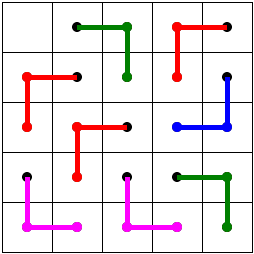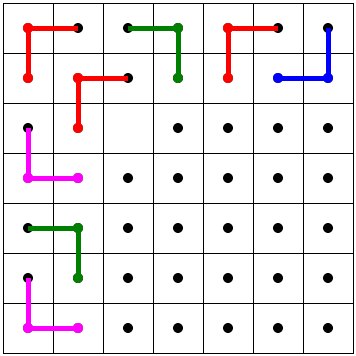# Tromino Puzzle: Deficient Squares

S. Golomb gave an inductive proof to the following fact: any 2n×2n board with one square removed can be tiled by right (or L-) trominoes - a piece formed by three adjacent squares in the shape of an L. Golomb's proof of the theorem became a model of elegance in elementary mathematics.

I-Ping Chu and Richard Johnsonbaugh extended Golomb's result to squares with sides not necessarily a power of 2. More accurately:

### Theorem

If n ≠ 5, then a deficient n×n board can be tiled with L-trominoes if and only if n is not a multiple of 3.

(The board is deficient if one of the small unit squares is missing.)

Some 5×5 deficient boards can be tiled, others cannot. Although 5 appears as an exceptional case in the theorem, the 5×5 board with a removed corner can be tiled and plays an important role in the proof. The proof does exploits other special cases.

The applet below helps you test your understanding of the theorem by tiling the board manually. It takes three clicks to place a tromino piece on the board: click on three adjacent squares in sequence. (Do not drag the mouse.)

### This applet requires Sun's Java VM 2 which your browser may perceive as a popup. Which it is not. If you want to see the applet work, visit Sun's website at https://www.java.com/en/download/index.jsp, download and install Java VM and enjoy the applet.

 What if applet does not run?

Note that for small (2×2 and 4×4) boards the solution is unique and follows Golomb's proof. For larger boards, viz. starting with 8×8, this is no longer true: there is a good deal of solutions. However, some thinking is still required to tile the board and, more often than not, careless tiling will produce 1 and 2 squares pockets.

Another applet provides additional insight into the tiling with L-trominoes.

Interstingly, we run into an entirely different situation if we try to cover the chessboard with straight trominos. Now, we'll have to consider very carefully which single square may or may not be removed!

### References

1. I-Ping Chu, Richard Johnsonbaugh, Tiling Deficient Boards with Trominoes, Mathematics Magazine, Vol. 59, No. 1 (Feb., 1986), pp. 34-40• Covering A Chessboard With Domino
• Dominoes on a Chessboard
• Tiling a Chessboard with Dominoes
• Vertical and Horizontal Dominoes on a Chessboard
• Straight Tromino on a Chessboard
• Golomb's inductive proof of a tromino theorem
• Tromino Puzzle: Interactive Illustration of Golomb's Theorem
• Tromino as a Rep-tile
• Tiling Rectangles with L-Trominoes
• Squares and Straight Tetrominoes
• Covering a Chessboard with a Hole with L-Trominoes
• Tiling a Square with Tetrominoes Fault-Free
• Tiling a Square with T-, L-, and a Square Tetrominoes
• Tiling a Rectangle with L-tetrominoes
• Tiling a 12x12 Square with Straight Trominoes
• Bicubal Domino
•### Theorem

If n ≠ 5, then a deficient n×n board can be tiled with L-trominoes if and only if n is not a multiple of 3.

The proof is a consequences of several partial results.

### Proposition 1

A 5×5 board with one corner square removed can be tiled

The proof is by inspection:Observe that the board with any of the squares next to a corner one removed could not be tiled with L-trominoes.

### Proposition 2

A (2i)×(3j) board, i, j > 1, can be tiled.

Such a board could be tiled by 2×3 rectangles each composed of 2 trominoes:More general rectangles can be tiled with L-trominoes, but the above is the only case used below.

### Proposition 3

Every deficient 7×7 board can be tiled.

The proof is again by inspection. There are just a few cases to consider. Enumerate squares starting in the upper left corner of the board with two integers (row, column). Because of the symmetry, we only have to check the cases of missing squares for 1 ≤ row ≤ column ≤ 4.The untiled portion of the board is the deficient 5×5 board from Proposition 1 and can be tiled. If we enumerate squares starting in the upper left corner of the board with two integers (row, column), the diagram tells us that the 7×7 board with the (3, 3) removed could be tiled. With a slight modification it also shows how to tile the board with squares (2, 2), (3, 2), (2, 3) removed.This diagram serves to show tilings with one of the squares - (1, 1), (1, 2), (2, 2), (2, 1) - removed.This diagram serves to show tilings with one of squares - (1, 3), (2, 3), (1, 4), (2, 4) - removed.

Two additional tilings (without (4, 4) and (3, 4) squares) complete the proof:### Proposition 4

Any deficient n×n board, with n is odd, n > 5, and not divisible by 3, can be tiled by L-trominoes.

First, we examine a deficient 11×11 board. As the diagram suggests, the board can be split into the a 7×7 piece (that is assumed to contain the missing square), two 4×6 pieces, and one 5×5 piece, with one corner square removed.As it was already shown, all four can be tiled with L-trominoes.

From here we proceed by induction. Assume that n is odd, not divisible by 3, and greater than 11. For the inductive step, assume that the statement holds for all smaller odd n not divisible by 3.

Split the board as shown below. The (n - 6)×(n - 6) pieces is assumed to contain the missing square.If n is odd and not divisible by 3, so is (n - 6), making the (n - 6)×(n - 6) tilable by the inductive assumption. On the other hand, for n odd, n - 7 is even, so that the (n - 7) & times; 6 pieces can be tiled by Proposition 2. The deficient 7×7 pieces can, in turn, be tiled due to Proposition 3.

### Proposition 5

Any deficient n×n board, with n even, greater than 1 and not divisible by 3 can be tiled with L-trominoes.

We start the induction with the observation that the 2×2, 4×4, and 8×8 deficient boards can be tiled (either by inspection or from Golomb's theorem).

Assume n is even, greater than 8, and is not divisible by 3. Suppose also that all smaller boards with such n can be tiled. Split the board into 4 pieces as shown:We assume that the missing square is located in the (n - 3)×(n - 3) piece. If n is even and not divisible by 3, then (n - 3) is odd and not divisible by 3. Given that n > 8, n - 3 > 5 so that Proposition 4 applies. The (n - 4)×3 pieces can be tiled by Proposition 2. So the whole board can be tiled and we are done.

A combination of the 5 propositions proves the theorem:

If n ≠ 5, then a deficient n×n board can be tiled with L-trominoes if and only if n is not a multiple of 3.